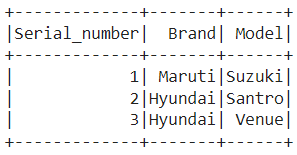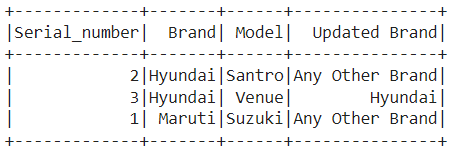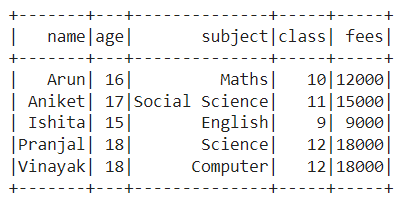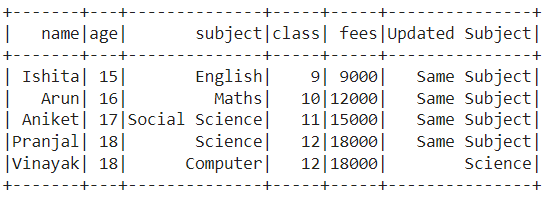Open in App
Not now

# PySpark lag() Function

• Last Updated : 30 Jan, 2023

The function that allows the user to query on more than one row of a table returning the previous row in the table is known as lag in Python. Apart from returning the offset value, the lag function also gives us the feature to set the default value in spite of None in the column. The setting of the default value is optional but it proves to b useful numerous times. In this article, we will discuss regarding the same, i.e., setting the default value for pyspark.sql.functions.lag to a value within the current row.

Syntax: pyspark.sql.functions.lag(data_frame[‘lag_column’], lag_value, ‘default_value’)

Here,

• lag_column: It is the column on which lag has to be done.
• lag_value: It is the value by which lag has to be performed on the particular column.
• default_value: It is the value that needs to be set inspite of None in the column.

### Example 1:

In this example, we created the data frame which has 3 columns ‘Serial_Number‘, ‘Brand‘ and ‘Model‘ as follows:Then, partition using the Window function on the Brand column and lag on the column Brand by 1 using lag function and set the default value ‘Any Other Brand‘ for rest of the cases.

## Python3

 `# PySpark - How to set the default ` `# value for pyspark.sql.functions ` `#.lag to a value within the current row? ` ` `  `# Import the SparkSession, Window and lag libraries ` `from` `pyspark.sql ``import` `SparkSession, Row ` `from` `pyspark.sql.window ``import` `Window ` `from` `pyspark.sql.functions ``import` `lag ` ` `  `# Create a spark session using getOrCreate() function ` `spark_session ``=` `SparkSession.builder.getOrCreate() ` ` `  `# Create a data frame using createDataFrame function ` `data_frame ``=` `spark_session.createDataFrame([Row(Serial_number``=``1``, ` `                                                ``Brand``=``'Maruti'``, ` `                                                ``Model``=``'Suzuki'` `                                               ``), ` `                                            ``Row(Serial_number``=``2``,  ` `                                                ``Brand``=``'Hyundai'``, ` `                                                ``Model``=``'Santro'` `                                               ``),  ` `                                            ``Row(Serial_number``=``3``, ` `                                                ``Brand``=``'Hyundai'``, ` `                                                ``Model``=``'Venue'``)]) ` ` `  `# Partition data through Brand column and sort it ` `# through Brand column in Window function ` `Windowspec ``=` `Window.partitionBy(``"Brand"``).orderBy(``"Brand"``) ` ` `  `# Calculating lag of brand by 1 for each student and using default ` `# value 'Any Other Brand' and putting in new column 'Updated Brand' ` `data_frame.withColumn(``'Updated Brand'``, lag( ` `    ``data_frame[``'Brand'``], ``1``, ``'Any Other Brand'``).over(Windowspec)).show() `

Output:### Example 2:

In this example, we have used a data set (link), which is basically a 5×5 data frame as follows:Then, partition on age, class and fees columns using the Window function and lag on the column subject by 1 using the lag function and set the default value ‘Same Subject‘ for rest of the cases.

## Python3

 `# PySpark - How to set the default value ` `# for pyspark.sql.functions.lag to ` `# a value within the current row? ` ` `  `# Import the SparkSession, Window and lag libraries ` `from` `pyspark.sql ``import` `SparkSession ` `from` `pyspark.sql.window ``import` `Window ` `from` `pyspark.sql.functions ``import` `lag ` ` `  `# Create a spark session using getOrCreate() function ` `spark_session ``=` `SparkSession.builder.getOrCreate() ` ` `  `# Read the CSV file ` `data_frame ``=` `csv_file ``=` `spark_session.read.csv( ` `    ``'/content/student_data.csv'``, sep``=``','``, inferSchema``=``True``, header``=``True``) ` ` `  `# Declare a list according to which partition has to be done ` `column_list ``=` `[``"age"``, ``"class"``, ``"fees"``] ` ` `  `# Partition data through list elements and sort it ` `# through age column in Window function ` `Windowspec ``=` `Window.partitionBy(column_list).orderBy(``"age"``) ` ` `  `# Calculating lag of subject by 1 for each student ` `# and putting in new column 'Updated Subject' ` `data_frame.withColumn(``'Updated Subject'``, lag( ` `    ``data_frame[``'subject'``], ``1``, ``'Same Subject'``).over(Windowspec)).show() `

Output:My Personal Notes arrow_drop_up
Related Articles